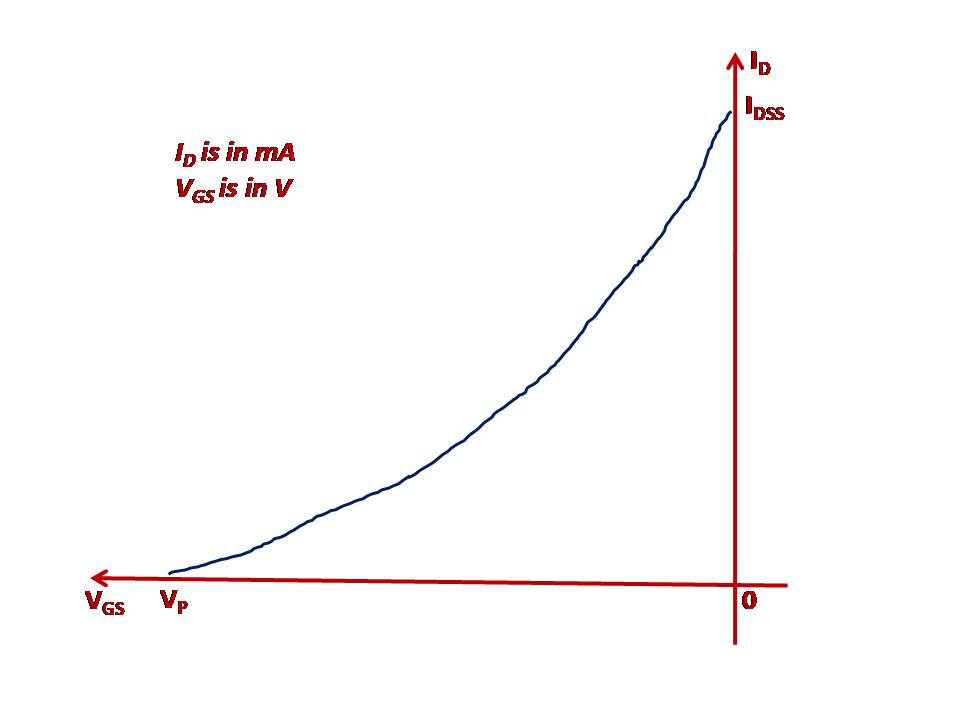### Mutual or Transfer Characteristic of n-channel JFET

Mutual or Transfer Characteristic of n-channel JFET:

This is a curve that is plotted between the ID and VGS (negative for n-channel JFET and positive for p-channel JFET) for a constant value of VDS.

The curve is plotted by varying the value of VGS and finding the value of the ID. The Figure 9 shows the Transfer characteristic of the n-channel JFET.Figure 9: The Transfer Characteristics of the N-Channel JFET

From these characteristics we can calculate a parameter called mutual conductance (gm). As is known the parameter mutual conductance is a value which identifies the relationship between the output parameter (current) and the input value (Voltage).

The equation for the parameter gm is given by:

gm=IDVGS

It may be noted here that the voltage VDS is kept constant at a certain value, say 6V.

Note 7:

It can be observed from the Figure 9 above that complete channel cutoff is possible here, because the channel cutoff is due to the VGS applied and not because of the Drain current where the VGS is 0.